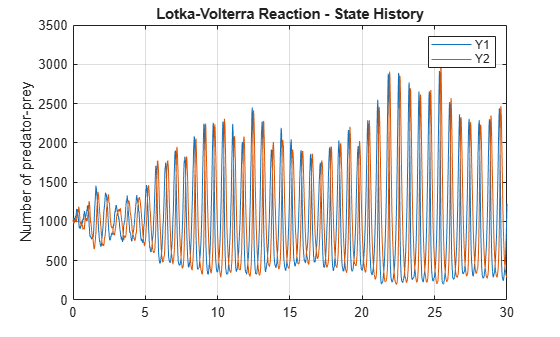Documentation

## Stochastic Simulation of the Lotka-Volterra Reactions

This example shows how to build and simulate a model using the SSA stochastic solver.

The following model will be constructed and stochastically simulated:

• Reaction 1: x + y1 -> 2 y1 + x, with rate constant, c1 = 10.

• Reaction 2: y1 + y2 -> 2 y2, with rate constant, c2 = 0.01.

• Reaction 3: y2 -> z, with rate constant, c3 = 10.

• Initial conditions: x=1 (constant), y1=y2=1000, z=0.

• Note: Species 'x' in Reaction 1 is represented on both sides of the reaction to model the assumption that the amount of x is constant.

These reactions can be interpreted as a simple predator-prey model if one considers that the prey population (y1) increases in the presence of food (x) (Reaction 1), that the predator population (y2) increases as they eat prey (Reaction 2), and that predators (y2) die of natural causes (Reaction 3).

This example uses parameters and conditions as described in Daniel T. Gillespie, 1977, "Exact Stochastic Simulation of Coupled Chemical Reactions," The Journal of Physical Chemistry, vol. 81, no. 25, pp. 2340-2361.

### Register Units for the Model

```sbioaddtolibrary(sbiounit('rabbit','molecule',1)); sbioaddtolibrary(sbiounit('coyote','molecule',1)); sbioaddtolibrary(sbiounit('food','molecule',1)); sbioaddtolibrary(sbiounit('amountDimension','molecule',1));```

### Create the Lotka-Volterra Model

```model = sbiomodel('Lotka-Volterra Model'); c = addcompartment(model,'C'); c.CapacityUnits = 'meter^3';```

### Add Reaction 1 to the Model Object

`r1 = addreaction(model,'x + y1 -> 2 y1 + x')`
``` SimBiology Reaction Array Index: Reaction: 1 x + y1 -> 2 y1 + x ```
```% Set the Kinetic Law for Reaction 1. kl1 = addkineticlaw(r1, 'MassAction'); % Add rate constant parameter, c1, to reaction with value = 10 p1 = addparameter(kl1, 'c1', 'Value', 10); kl1.ParameterVariableNames = {'c1'}; % Add units to c1 p1.ValueUnits = '1/(second*rabbit)'; % Set initial amounts for species in Reaction 1 r1.Reactants(1).InitialAmount = 1; % x r1.Reactants(2).InitialAmount = 1000; % y1 % Set the initial amount units for species in Reaction 1 r1.Reactants(1).InitialAmountUnits = 'food'; % x r1.Reactants(2).InitialAmountUnits = 'rabbit'; % y1```

### Add Reaction 2 to the Model Object

`r2 = addreaction(model,'y1 + y2 -> 2 y2')`
``` SimBiology Reaction Array Index: Reaction: 1 y1 + y2 -> 2 y2 ```
```% Set the kinetic law for Reaction 2. kl2 = addkineticlaw(r2, 'MassAction'); % Add rate constant parameter, c2, to kinetic law with value = 0.01 p2 = addparameter(kl2, 'c2', 'Value', 0.01); kl2.ParameterVariableNames = {'c2'}; % Add units to c2 p2.ValueUnits = '1/(second*coyote)'; % Set initial amounts for new species in Reaction 2 r2.Products(1).InitialAmount = 1000; % y2 % Set the initial amount units for new species in Reaction 2 r2.Products(1).InitialAmountUnits = 'coyote'; % y2```

### Add Reaction 3 to the Model Object

`r3 = addreaction(model,'y2 -> z')`
``` SimBiology Reaction Array Index: Reaction: 1 y2 -> z ```
```% Add "bogus" units to trash variable 'z' r3.Products(1).InitialAmountUnits = 'amountDimension'; % Set the kinetic law for Reaction 3. kl3 = addkineticlaw(r3, 'MassAction'); % Add rate constant parameter, c3, to reaction with value = 10 p3 = addparameter(kl3, 'c3', 'Value', 10); kl3.ParameterVariableNames = {'c3'}; % Add units to c3 p3.ValueUnits = '1/second';```

### Display the Completed Model Objects

`model`
``` SimBiology Model - Lotka-Volterra Model Model Components: Compartments: 1 Events: 0 Parameters: 3 Reactions: 3 Rules: 0 Species: 4 ```

### Display the Reaction Objects

`model.Reactions`
``` SimBiology Reaction Array Index: Reaction: 1 x + y1 -> 2 y1 + x 2 y1 + y2 -> 2 y2 3 y2 -> z ```

### Display the Species Objects

`model.Species`
``` SimBiology Species Array Index: Compartment: Name: InitialAmount: InitialAmountUnits: 1 C x 1 food 2 C y1 1000 rabbit 3 C y2 1000 coyote 4 C z 0 amountDimension ```

### Simulate with the Stochastic (SSA) Solver & Plot

```cs = getconfigset(model,'active'); cs.SolverType = 'ssa'; cs.StopTime = 30; cs.SolverOptions.LogDecimation = 200; cs.CompileOptions.UnitConversion = true; [t,X] = sbiosimulate(model); plot(t, X(:,2), t, X(:,3)); legend('Y1', 'Y2'); title('Lotka-Volterra Reaction - State History'); ylabel('Number of predator-prey'); grid on;```### Show Phase Portrait of Y1 to Y2

```plot(X(:,2),X(:,3)); title('Lotka-Volterra Reaction - Y1 vs. Y2'); xlabel('Number of Y1 rabbits'); ylabel('Number of Y2 coyotes');``````% Clean up units. sbioremovefromlibrary('unit', 'rabbit'); sbioremovefromlibrary('unit', 'coyote'); sbioremovefromlibrary('unit', 'food'); sbioremovefromlibrary('unit', 'amountDimension');```
##### SupportGet trial now